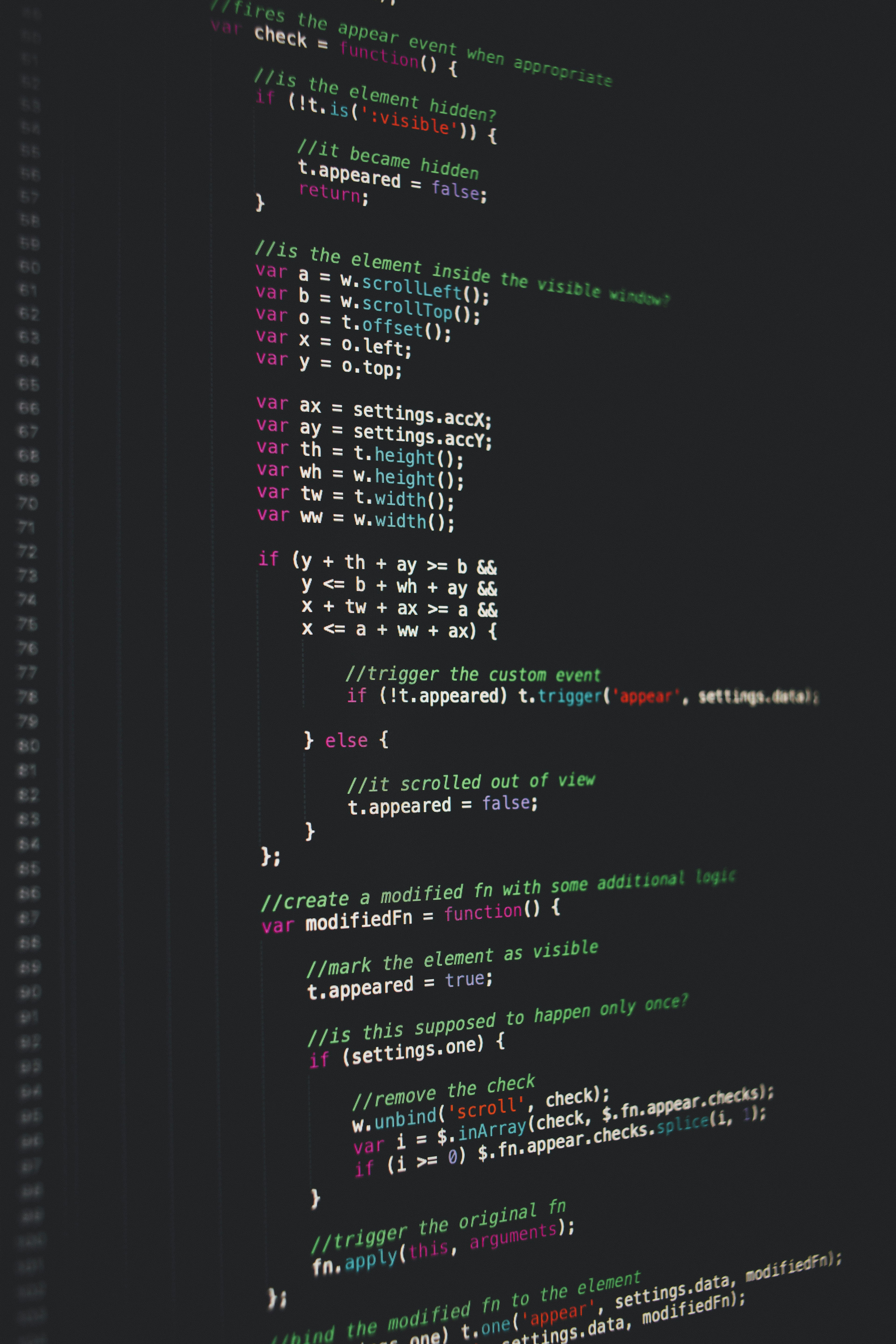Which programming language to choose as a beginner?

# Which programming language to choose as a beginner?

As someone who have just planned to enter into programming world, we quite often get confused which programming language to learn or start with because of the availability of so many different programming languages.My friend Levin, a beginner to programming, is so confused about which programming language to start with. For the past few days he has been jumping from learning javascript to learning python, c++, java.

But as a beginner in programming you shouldn't be so confused. To keep it simple, learn one language and master it. Then the next one is going to be as easy as pie.

The only way to learn a new programming language is by writing programs in it.

In the rest of the article, I will show you how to write a code to reverse a string in 5 popular programming languages.

By reversing a string I mean. Turning something like this.

Cat Meow

To this

woeM taC

Here’s how to reverse a string in five popular programming languages.

#### Reversing a string in C++

// A Simple C++ program to reverse a string
#include <bits/stdc++.h>
using namespace std;
// Function to reverse a string
void reverseStr(string& str)
{
int n = str.length();
// Swap character starting from two
// corners
for (int i = 0; i < n / 2; i++)
swap(str[i], str[n - i - 1]);
}
// Driver program
int main()
{
string str = "medium.com";
reverseStr(str);
cout << str;
return 0;
}

Doesn't make any sense? Don’t worry. The next one is easy.

#### Reversing a string in Python

txt = "Hello World" [::-1]
print(txt)

Easy as pie. We just have to add [::-1] to reverse a string in python.

#### Reversing a String in Java

import java.util.Scanner;
public class ReverseString
{
public static void main(String[] args)
{
System.out.println("Enter string to reverse:");

String reverse = "";

for(int i = str.length() - 1; i >= 0; i--)
{
reverse = reverse + str.charAt(i);
}

System.out.println("Reversed string is:");
System.out.println(reverse);
}
}

This looks difficult

#### Reversing a string in Ruby

puts "GeeksforGeeks".reverse

Again quite easy. I would definitely choose Ruby or Python as a beginner.

#### Reversing a String in Javascript

function reverseString(str) {
return str.split("").reverse().join("");
}
reverseString("hello");

Easy? Hard? Can’t say for sure.

Now you have seen all the different ways to reverse a string in 5 popular programming languages.

You might be wondering why the code in C++ & Java is much longer than the rest.

The answer is simple, in C++ or Java, you are writing an algorithm to reverse a string and also using the algorithm in action. In the rest of the languages, you are using a built-in method.

Let’s try to understand this by taking an example from mathematics.

An arithmetic progression is a sequence where each term is a certain number larger than the previous term. The terms in the sequence are said to increase by a common difference, d.

For example: 3, 5, 7, 9, 11, is an arithmetic progression where d = 2. The nth term of this sequence is 2n + 1.

And we know that the sum to n terms of an arithmetic progression is given by:

Sn = ½ n [ 2a + (n - 1)d ]

Now using the above formula, you can easily calculate the sum of an arithmetic progression. But if you are told to derive the above formula as well it will be much longer.

It is derived as follows

The sum to n terms is given by:
Sn = a + (a + d) + (a + 2d) + … + (a + (n – 1)d)     (1)
If we write this out backwards, we get:
Sn = (a + (n – 1)d) + (a + (n – 2)d) + … + a            (2)
Now let's add (1) and (2):
2Sn = [2a + (n – 1)d] + [2a + (n – 1)d] + … + [2a + (n – 1)d]
So Sn = ½ n [2a + (n – 1)d]

Similar to above, in C++ and Java you have to write your own formula when reversing a string. This gives us the flexibility to use our own formula or algorithm to achieve our desired task (here reversing a string). Also you can come up with the fastest algorithm to reverse a string and beat all the other languages in terms of speed. However it makes our code longer too.

On the other hand when using python, ruby, etc to reverse a string. You are using predefined functions. Which means, you are strictly using the algorithm defined by the creator or the team of those particular languages.

I wouldn’t go too deep in explaining the pros and cons of using each of the above languages. I would end this with small advice.

If you would like to understand all the core concepts of programming then start with C, C++, or Java. Sure, Python is cool, but remember it was created using C language.

And if you would like to quickly learn to do cool stuff, such as, creating a website, creating an app, task automation, etc. I would advise you to start with python or javascript.